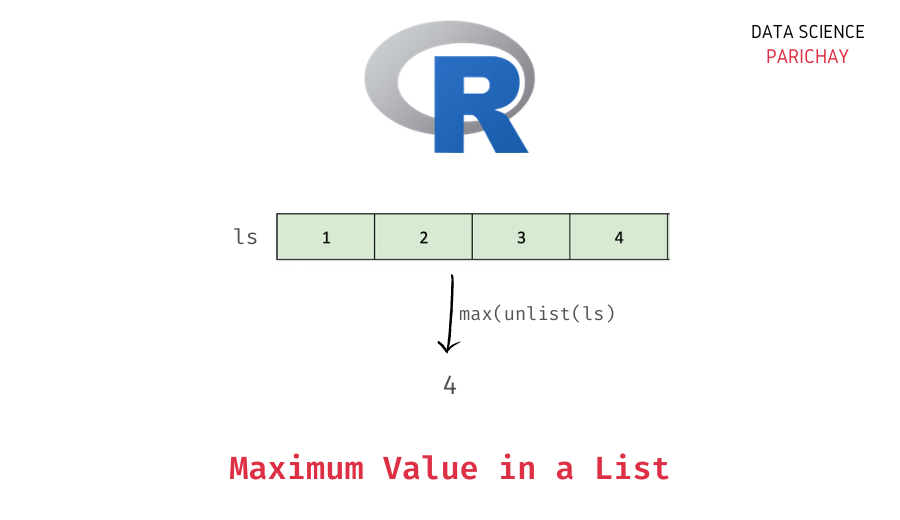# Get the Maximum Value in an R List

Lists are used in R to store one-dimensional data. Unlike vectors that can only store values of the same type, a list in R can store values of different types together. Although vectors are more commonly used in R, it can be handy to know some common operations on lists. In this tutorial, we will look at how to get the maximum value in an R list with the help of some examples.

## How to get the max value in a list in R?

You can use a combination of the `unlist()` function and the `max()` function in R to get the maximum value in a list. The following is the syntax –

```# get the max value in a list
max(unlist(ls), na.rm=FALSE)```

First, use the unlist() function to convert the list into a vector, and then use the max() function to get the maximum value. The following are the arguments that you can give to the `max()` function in R.

• x – The vector for which you want to compute the max value.
• na.rm – (Optional argument) Indicates whether to remove missing values before computing the maximum. It is `FALSE` by default.

The `max()` function returns the max value in the passed vector.

## Examples

Let’s look at some examples of using the above method to get the max value in a list.

### Maximum value in a list of numbers

First, let’s see what happens if we directly apply the `max()` function to a list without converting it to a vector.

```# create a list of numbers
ls <- list(1, 3, 5, 4)
# max value in list
max(ls)```

Output:

`Error in max(ls): invalid 'type' (list) of argument`

You can see that we get an error. This is because we cannot apply the `max()` function in R directly to a list.

📚 Data Science Programs By Skill Level

Introductory

Intermediate ⭐⭐⭐

🔎 Find Data Science Programs 👨‍💻 111,889 already enrolled

Disclaimer: Data Science Parichay is reader supported. When you purchase a course through a link on this site, we may earn a small commission at no additional cost to you. Earned commissions help support this website and its team of writers.

Now let’s use the above syntax, that is, first convert the list to a vector using `unlist()` and then apply the `max()` function.

```# create a list of numbers
ls <- list(1, 3, 5, 4)
# max value in list
max(unlist(ls))```

Output:

`5`

We get 5 as the maximum value in the above list, which is the correct answer.

What would happen if there are some `NA` present values in the list?

Let’s find out.

First, we will create a list with some `NA` values and then apply the same syntax as above without any additional arguments.

```# create a list with NA values
ls <- list(1, 3, NA, 5, NA, 4, NA)
# max value in the list
max(unlist(ls))```

Output:

`<NA>`

You can see that we get `NA` as the output. This is because comparing a value with NA results in an `NA` in R.

### Maximum in a list with `NA` values

You can pass `TRUE` to the `na.rm` parameter of the `max()` function to exclude missing values when computing the maximum value in a vector. This will ignore the `NA` values and compute the maximum from the remaining values.

```# create a list with NA values
ls <- list(1, 3, NA, 5, NA, 4, NA)
# max value in the list
max(unlist(ls), na.rm=TRUE)```

Output:

`5`

Now we get the maximum value in the above list as 5.

### Maximum value in a character list

The above syntax also works similarly for a list of characters. For example, let’s see what we get on applying it to a list of characters.

```# create a list of chracters
ls <- list("a", "b", "c", "d")
# max value in the list
max(unlist(ls))```

Output:

`'d'`

Here, we get ‘d’ as the maximum value in the above list which contains the values ‘a’, ‘b’, ‘c’, and ‘d’.

You might also be interested in –

•